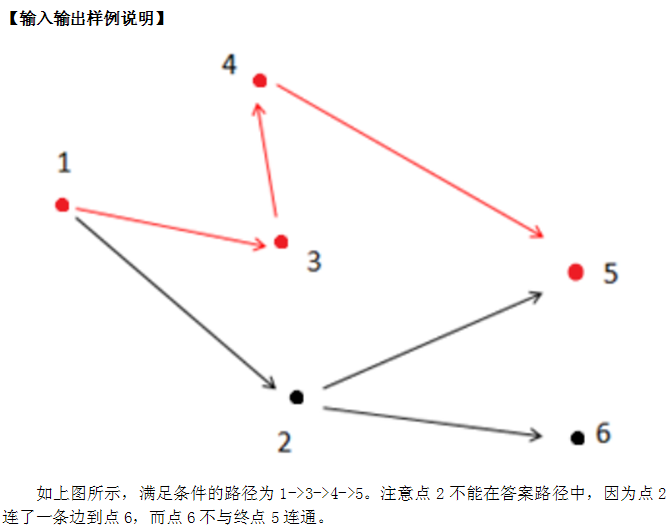# NOIp2014提高组 P2296 寻找道路 题解【图论】【最短路】【搜索】

思路简单但是细节很多的一道题。

## 题目描述 Description

1．路径上的所有点的出边所指向的点都直接或间接与终点连通。

2．在满足条件1的情况下使路径最短。

## 输出描述 Output Descriptionroad.in road.out 6 6 1 2 1 3 2 6 2 5 4 5 3 4 1 5 3## 题解：

首先要满足的一个条件是只能经过能出边指向的点能独立到达终点的点，因此一开始反向BFS一遍，找出能到达的点做标记，前向星的奇偶性可以完成这一点。

然后对所有上述能到达的点的出边进行遍历，如果找到一条边指向的点不能到达终点，那么当前点就不能在路径上。不过根据题意，如果当前点可以到终点，那么指向当前点的其他点是不受这个点影响的。所以我们把走不到终点的点标记为-1，能走到终点但是指向点有-1的点标记为0，完全符合要求的置为1。这样不仅可以只保留1，而且方便了1部分的更新。

这个题的思路比较好想，细节还是很多的。

## Code：

#include<cstdio>
#include<cstring>
#include<queue>
using std::deque;
struct edge
{
int n,nxt;
edge(int n,int nxt)
{
this->n=n;
this->nxt=nxt;
}
edge()
{
nxt=-1;
}
}e;
{
}
int ava;
int dis,used;
void spfa(int s)
{
deque<int> q;
memset(used,0,sizeof(used));
memset(dis,0x3f,sizeof(dis));
dis[s]=0;
used[s]=1;
q.push_back(s);
while(!q.empty())
{
int k=q.front();
q.pop_front();
used[k]=0;
if((!(i&1))&&ava[e[i].n]==1)
if(dis[e[i].n]>dis[k]+1)
{
dis[e[i].n]=dis[k]+1;
if(!used[e[i].n])
{
used[e[i].n]=1;
if(q.empty()||dis[e[i].n]<=dis[q.front()])
q.push_front(e[i].n);
else
q.push_back(e[i].n);
}
}

}
}
int main()
{
int n,m,u,v;
scanf("%d%d",&n,&m);
for(int i=1;i<=m;i++)
{
scanf("%d%d",&u,&v);
}
int s,t;
scanf("%d%d",&s,&t);
int q,l=0,r=0;
q[++r]=t;
used[t]=1;
while(l<r)//首先bfs找出可以used的
{
int p=q[++l];
if(i&1&&!used[e[i].n])//i&1表示是反向边
{
q[++r]=e[i].n;
used[e[i].n]=1;
}
}
for(int i=1;i<=n;i++)//不能到终点的置为-1
if(!used[i])
ava[i]=-1;
l=0,r=0;
q[++r]=t;
memset(used,0,sizeof(used));
used[t]=1;
while(l<r)//反向遍历
{
int p=q[++l];
if(i&1&&ava[e[i].n]==0&&!used[e[i].n])
q[++r]=e[i].n,used[e[i].n]=1;
int flag=-1;
if((i&1)==0&&ava[e[i].n]==-1)
{
flag=0;
break;//要有break
}
else
flag=1;
ava[p]=flag;
}
spfa(s);
if(dis[t]==0x3f3f3f3f)
puts("-1");
else
printf("%d\n",dis[t]);
return 0;
}

### 1 说点什么0 Followers

Most reacted comment
0 Comment authors
Recent comment authors
SubscribewpDiscuz